# mnist手写数字识别（TensorFlow-GPU）-----------原理及代码

1. 导入数据集。
2. 分析mnist样本特点定义变量。
3. 构建模型。
4. 训练模型并输出中间状态参数。
5. 测试模型。
6. 保存模型。
7. 读取模型。

### 一、导入手写图片的数据集

（1） mnist数据集

mnist数据集里面包含各种手写数字图片，如下图一所示：

它包含每一张图片对应的标签，告诉我们这个是数字几，并对下面的四张图片打上标签5,0,4,1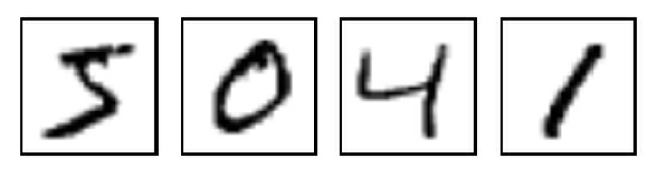（2） 利用TensorFlow代码下载mnist数据集

通过TensorFlow所提供的库，进行下载minst数据集：

# -*- coding: utf-8 -*-
# !/usr/bin/env python
# @Time    : 2019/5/17 17:03
# @Author  : xhh
# @Desc    :  minist数据集下载
# @File    : mnist_data_load.py
# @Software: PyCharm
from tensorflow.examples.tutorials.mnist import  input_data
import pylab

mnist = input_data.read_data_sets("MINST_daya/", one_hot=True)
print("输入数据：",mnist.train.images)
print("数据的shape：",mnist.train.images.shape)

# 展示数据集中的一张图片
im = mnist.train.images
im = im.reshape(-1,28)
pylab.imshow(im)
pylab.show()

onn_hot编码：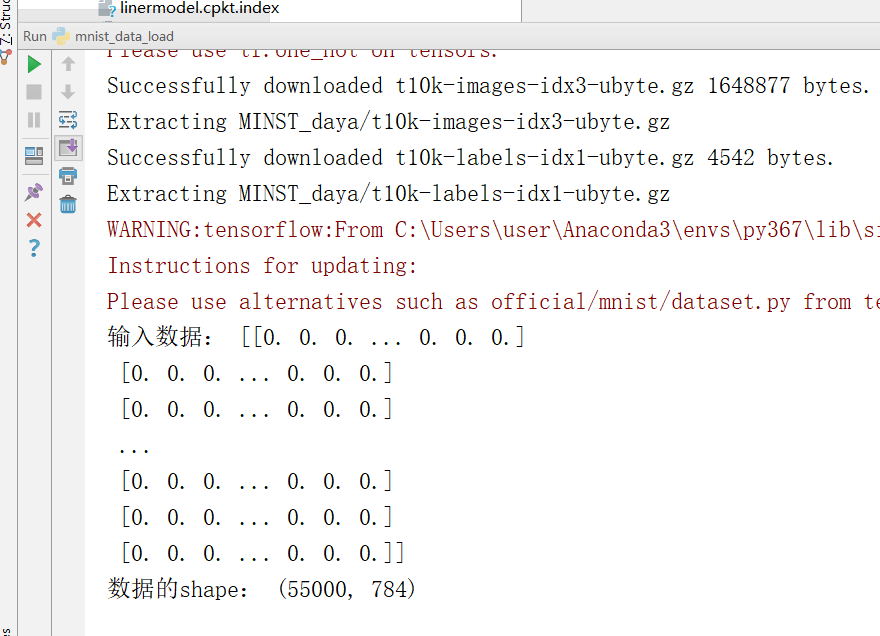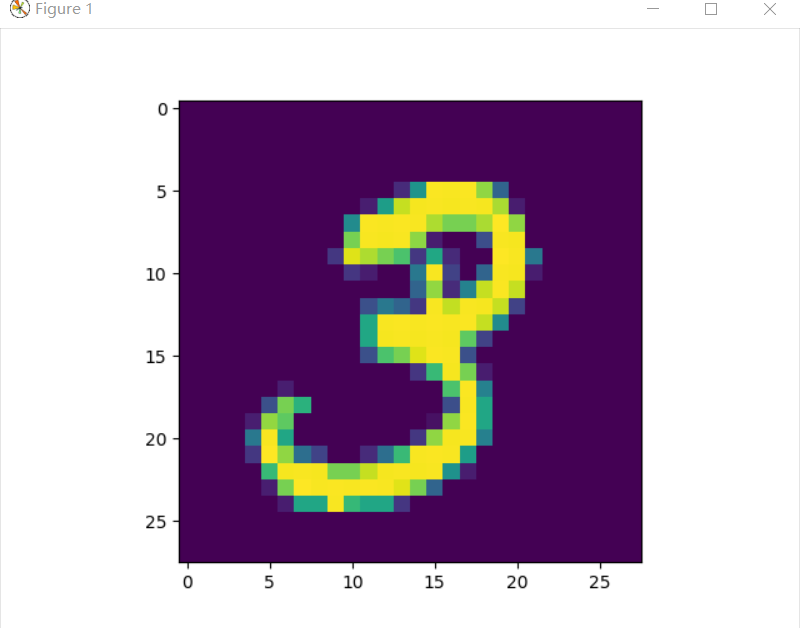图二

mnist数据集如上图二的彩色图片所示，为彩色图片，它是3通道的，由RGB(红、黄、蓝)构成，图一的是黑白的是单通道图片，数值为0~255之间的数字，代表其颜色的深度。

（3）mnst数据集的组成

在MNIST训练数据集中，mnist.train.images是一个形状为[55000，784]的张量。其中，第1个维度数字用来索引图片，第2个维度数字用来索引

MNIST里包含3个数据集：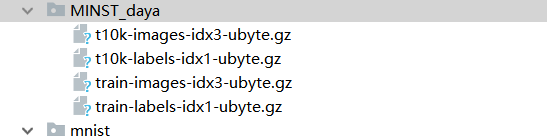### 二、分析图片的特点，定义变量

由于输入图片是个550000×784的矩阵，所以先创建一个[None，784]的占位符x和一个[None，10]的占位符y，然后使用feed机制将图片和标签

from tensorflow.examples.tutorials.mnist import  input_data
import pylab
import tensorflow as tf

mnist = input_data.read_data_sets("MINST_daya/", one_hot=True)

tf.reset_default_graph()

# 定义占位符
x = tf.placeholder(tf.float32, [None, 784])  # mnist data 维度28*28=784
y = tf.placeholder(tf.float32, [None, 10])  #0-9 数字 ==>10class

在定义占位符时，x和y中的None,表示此张量的第一个维度可以是任何长度的。x代表能够输入任意数量的mnist图像，每张图可以展成784维的向量。

### 三、构建模型

（1） 定义学习参数

在TensorFlow里，使用Variable来定义学习参数。模型也需要权重值和偏置量，它们被统一叫做学习参数一个Variable代表一个可修改的张量，定义在TensorFlow的图（一个执行任务）中，其本身也是一种变量。使用Variable定义的学习参数可以用于计算输入值，也可以在计算中被修改。


# 定义学习参数
# 设置模型的权重
W = tf.Variable(tf.random_normal([784, 10]))  # W的维度是[784, 10]
b = tf.Variable(tf.zeros())


(2)  定义输出节点


# 定义输出节点， 构建模型
pred = tf.nn.softmax(tf.matmul(x, W) + b)  # softmax分类

首先，用tf.matmul（x，W）表示x乘以W，这里x是一个二维张量，拥有多个输入。然后再加上b，把它们的和输入tf.nn.softmax函数里。至此就构建好了正向传播的结构。也就是表明，只要模型中的参数合适，通过具体的数据输入，就能得到我们想要的分类。

（3） 定义反向传播结构


# 定义反向传播的结构，编译训练模型，得到合适的参数
cost = tf.reduce_mean(-tf.reduce_sum(y*tf.log(pred), reduction_indices=1))

# 参数设置
learning_rate = 0.01
# 使用梯度下降优化器
optimizer = tf.train.GradientDescentOptimizer(learning_rate).minimize(cost)


（1）将生成的pred与样本标签y进行一次交叉熵的运算，然后取平均值。
（2）将这个结果作为一次正向传播的误差，通过梯度下降的优化方法找到能够使这个误差最小化的b和W的偏移量。
（3）更新b和W，使其调整为合适的参数。整个过程就是不断地让损失值（误差值cost）变小。因为损失值越小，才能表明输出的结果跟标签数据越相近。当cost小到我们的需求时，这时的b和W就是训练出来的合适值。

（4） 训练模型并输出中间状态参数，并且进行模型的保存与测试

training_epochs = 25   # 将整个训练样本迭代25次
batch_size = 100    # 在训练过程中每次随机抽取100条数据进行训练
display_step = 1   # 迭代的步数
saver = tf.train.Saver()
model_path = "mnist/521model.ckpt"


# 开始训练
with tf.Session()  as sess:
# 初始化节点
sess.run(tf.global_variables_initializer())

# 启动循环开始训练
for epoch in range(training_epochs):
avg_cost = 0.
total_batch = int(mnist.train.num_examples/batch_size)
# 遍历全部的数据集
for i in range(total_batch):
batch_xs, batch_ys = mnist.train.next_batch(batch_size)
# 运行和优化节点的损失函数值
_, c = sess.run([optimizer, cost], feed_dict={x:batch_xs,
y: batch_ys})
# 计算平均损失值
avg_cost += c / total_batch

# 显示训练中的详细信息
if (epoch+1) % display_step ==0:
print("Epoch:","%04d"%(epoch+1), "cost=",'{:.9f}'.format(avg_cost))

print("训练成功！！")

# 模型测试
correct_prediction = tf.equal(tf.argmax(pred, 1), tf.argmax(y, 1))
# 计算准确率
accuracy = tf.reduce_mean(tf.cast(correct_prediction, tf.float32))
print("准确度：",accuracy.eval({x:mnist.test.images, y:mnist.test.labels}))

# 保存模型的权重
save_path = saver.save(sess, model_path)
print("模型文件在：%s"%save_path)


Epoch: 0001 cost= 8.658398746
Epoch: 0002 cost= 4.599675331
Epoch: 0003 cost= 3.098299387
Epoch: 0004 cost= 2.414841038
Epoch: 0005 cost= 2.031551510
Epoch: 0006 cost= 1.787429208
Epoch: 0007 cost= 1.617599975
Epoch: 0008 cost= 1.491779541
Epoch: 0009 cost= 1.394358738
Epoch: 0010 cost= 1.316281419
Epoch: 0011 cost= 1.251967654
Epoch: 0012 cost= 1.197913221
Epoch: 0013 cost= 1.151722029
Epoch: 0014 cost= 1.111743248
Epoch: 0015 cost= 1.076424035
Epoch: 0016 cost= 1.045415161
Epoch: 0017 cost= 1.017401275
Epoch: 0018 cost= 0.992323116
Epoch: 0019 cost= 0.969426456
Epoch: 0020 cost= 0.948599738
Epoch: 0021 cost= 0.929346439
Epoch: 0022 cost= 0.911827402
Epoch: 0023 cost= 0.895336545
Epoch: 0024 cost= 0.880129020
Epoch: 0025 cost= 0.865876571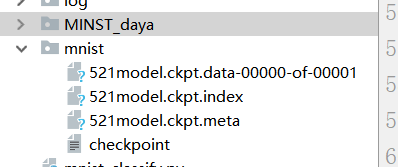# 读取模型
print("启动第二次session")
with tf.Session() as sess2:
# 初始化参数
sess2.run(tf.global_variables_initializer())
#从保存的模型中获取权重
saver.restore(sess2, model_path)

# 测试 model
correct_prediction = tf.equal(tf.argmax(pred, 1), tf.argmax(y, 1))
# 计算准确率
accuracy = tf.reduce_mean(tf.cast(correct_prediction, tf.float32))
print("准确度：",accuracy.eval({x:mnist.test.images, y:mnist.test.labels}))

output = tf.argmax(pred, 1)
batch_xs, batch_ys = mnist.train.next_batch(2)
outputval, predv = sess2.run([output, pred], feed_dict={x:batch_xs})
print(outputval, pred, batch_ys)

im = batch_xs
im = im.reshape(-1, 28)
pylab.imshow(im)
pylab.show()

im = batch_xs
im = im.reshape(-1, 28)
pylab.imshow(im)
pylab.show()


[0 2] Tensor("Softmax:0", shape=(?, 10), dtype=float32) [[1. 0. 0. 0. 0. 0. 0. 0. 0. 0.]
[0. 0. 1. 0. 0. 0. 0. 0. 0. 0.]]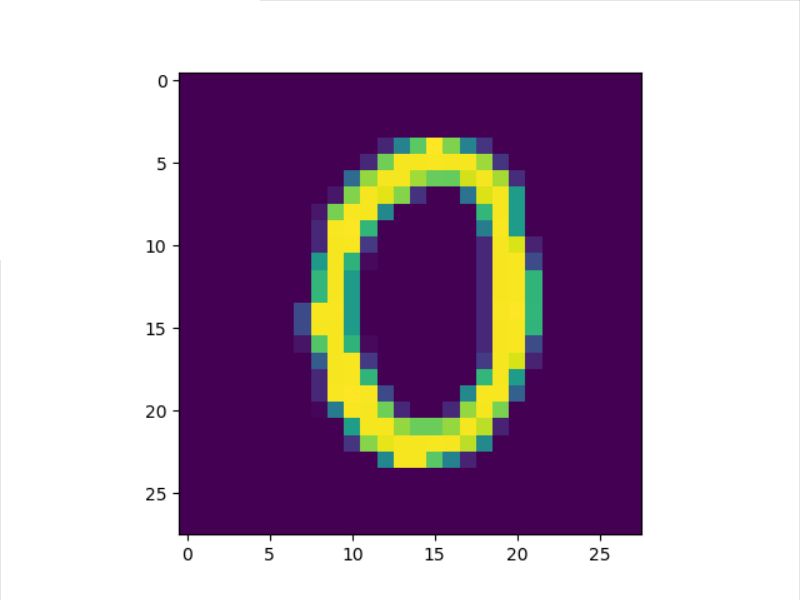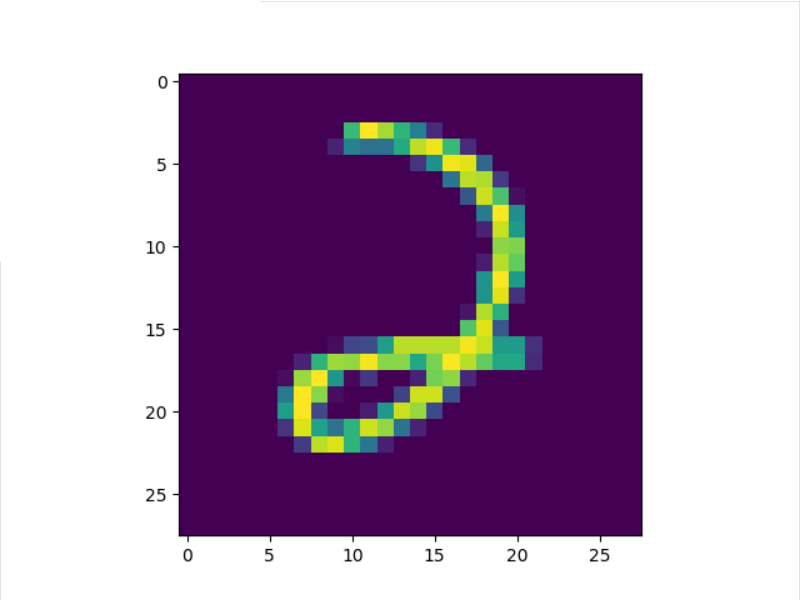04-092万+
11-082893
05-103万+
03-301258
09-111406
04-241万+
04-242400
03-14
09-07543
04-11
10-17
11-011350
11-076万+
04-286766
04-17450
©️2020 CSDN 皮肤主题: 创作都市 设计师:CSDN官方博客